Next: 9.4 Investigation of Fabricated Up: 9. Intrinsic Stress Effects Previous: 9.2 Sources of Intrinsic

# 9.3 Modeling of the Stress Sources

The goal of the modeling is to express the previous described microscopic stress phenomena with a macroscopic stress formulation for the respective source.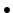Coalescence of Grain Boundaries:
The force which is generated by grain island impingement is(9.2)

where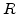is the radius of the hemispherical island (see Fig. 9.5a) and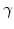is the surface energy of the contacting spheres.

The volume of material included in a hemispherical island upon coalescence is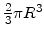. If the material in each island would spread uniformly over a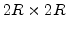square area on the substrate, the uniform depth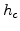would be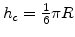. The area of a lateral face of this equivalent material block is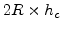, so that the uniform tensile stress on this surface which produces the same resultant force as in (9.2) is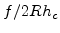or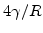. Therefore, the average intrinsic stress caused by coalescence in the film with thicknessis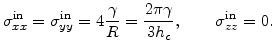(9.3)Misfit Stress:
Misfit stresses occur in crystalline films because of the lattice mismatch at the interface between film and substrate. If only the film lattice would adjust to the substrate lattice as demonstrated in Fig. 9.6b, the misfit strain in the film would be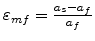. But in reality the lattice of the film as well as the substrate are both adapted at the interface, which is characterized by the misfit parameter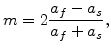(9.4)

where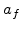and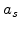are the lattice constants of the film and substrate (see Fig. 9.6a), respectively. The nonzero components of the misfit stress tensor are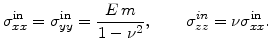(9.5)Grain Growth:
During grain growth some grain boundaries and their volumes disappear. Assume that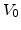is the pure crystal volume, where the excess volume of the grain boundaries is not included, and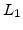is the average grain diameter. Then the grain boundary area per unit volume is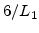for spherical grains. If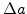is the excess volume per unit area of the grain boundary, the total excess volume for the grain boundaries in a volumeis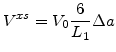(9.6)

The total grain volume is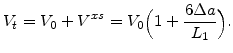(9.7)

The normalized volume change due to the disappearence of grain boundaries would be(9.8)

If the grain grows to a new diameter, the normalized volume change is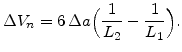(9.9)

As defined in (3.37) the strain components,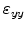, and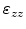are one third of the normalized volume change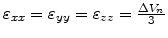. Therefore the intrinsic tensile stress associated with the grain growth is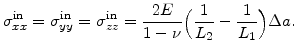(9.10)Excess Vacancy Annihilation:
The gaps at the grain boundaries are closed by stretching the grains. The stress calculation is in principle the same as for the grain growth. If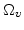is the vacancy volume and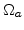is the atomic volume, then for a number of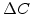vacancies which annihilate per unit volume, the normalized volume change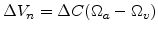. Therefore, the intrinsic stress caused by vacancy annihilation is given by(9.11)

Since during vacancy annihilation the vacancies diffuse to grain boundaries, the intrinsic stress can also be described with the more detailed and diffusion affiliated formulation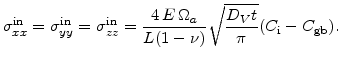(9.12)

Here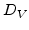is the vacancy diffusivity within the grain and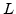is the grain diameter.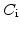is the vacancy concentration inside the grain and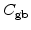in the grain boundary, respectively.Thermal Stress:
The developed intrinsic stress due to thermal mismatch in the film and in the substrate material is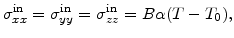(9.13)

where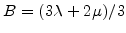is the bulk modulus with the Lame constants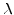and,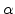is the thermal expansion coefficient, and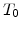is the ambient temperature.
For a uniform film the thermal stress is also uniform over the thickness. As in case of cantilevers, where after release still a part of the beam length is clamped at the bottom (see Fig. 9.2), this uniform stress has also a bending effect.Insertion of Excess Atoms:
The insertion of excess atoms into the grain boundaries creates a compressive stress in the film(9.14)

where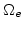is the volume of an excess atom and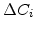is the number of excess atoms which are inserted per unit volume.

In this work a methodology to predict a qualitative strain or stress curve over the film thickness was found. This methodology is based on the knowledge of the different intrinsic stress sources, the observed deflection characteristic of the deposited thin film material, and human mind. In the state of the art of development this methodology can not weight the influence of the different single stress sources in the different film thicknesses automatically. In order to find the strain or stress curve automatically a more advanced thin film stress model is necessary. The development of a thin film model, which takes all stress sources into account and weights them for different film materials and process conditions automatically, should be done in future work.Next: 9.4 Investigation of Fabricated Up: 9. Intrinsic Stress Effects Previous: 9.2 Sources of Intrinsic

Ch. Hollauer: Modeling of Thermal Oxidation and Stress Effects